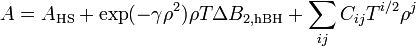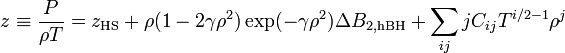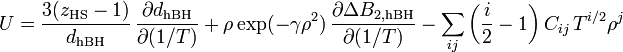# Difference between revisions of "Lennard-Jones equation of state"

The equation of state of the Lennard-Jones model.

## Johnson, Zollweg and Gubbins

Johnson, Zollweg and Gubbins  proposed an equation of state based on 33 parameters within a modified Benedict, Webb and Rubin equation of state, which accurately reproduces the vapour-liquid equilibrium curve.

## Kolafa and Nezbeda

The Kolafa and Nezbeda equation of state  provides us with the Helmholtz energy function: (Eq. 30):$A=A_{\mathrm{HS}} + \exp (-\gamma \rho^2) \rho T \Delta B_{2,{\mathrm{hBH}}} + \sum_{ij} C_{ij} T^{i/2} \rho^j$

the compressibility factor (Eq. 31)$z \equiv \frac{P}{\rho T}= z_{\mathrm{HS}} + \rho(1-2\gamma\rho^2) \exp (-\gamma \rho^2) \Delta B_{2,{\mathrm{hBH}}} + \sum_{ij} jC_{ij} T^{i/2-1} \rho^j$

and the internal energy (Eq. 32)$U= {3(z_{\rm HS}-1)\over d_{\rm hBH}}\, {\partial d_{\rm hBH}\over \partial (1/T)} + \rho \exp(-\gamma\rho^2)\,{\partial \Delta B_{\rm2,hBH}\over\partial (1/T)} - \sum_{ij} \left({i\over2}-1\right) C_{ij}\, T^{i/2} \rho^j$

On the following page is the FORTRAN code for the Kolafa and Nezbeda equation of state.

## Ree

The Ree equation of state  is an extension of the earlier work of Hansen  in the high temperature region.

## Boltachev and Baidakov

Boltachev and Baidakov have paid particular attention to including data from the metastable region .

## Pieprzyk-Brańka-Maćkowiak and Heyes

The Pieprzyk-Brańka-Maćkowiak and Heyes equation of state  consists of a parameterisation of the modified Benedict, Webb and Rubin equation of state.